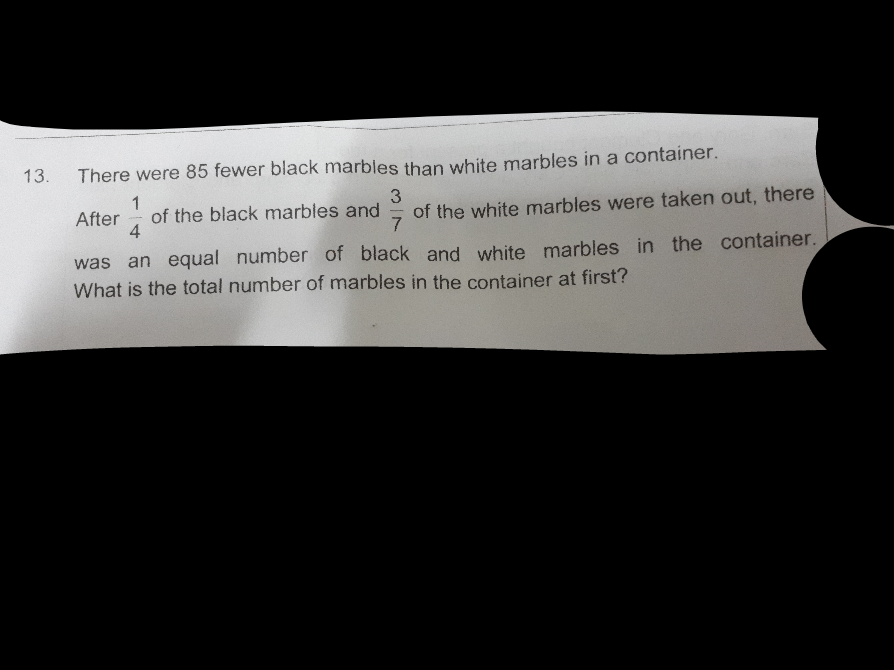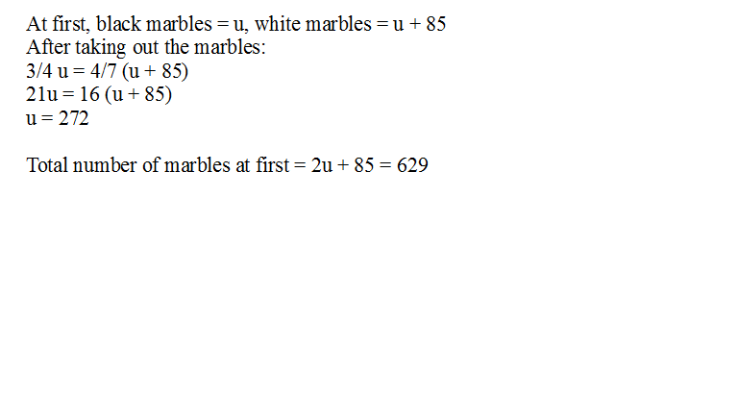# QuestionBelow is the method I will always propose for its “swiftness”… As long as 2 different fractions “are equal” (e.g. same amount taken out, same amount left, etc) – The foundation for this method is after kids should and have gone through much model drawing practices for showing 3 units =4 parts by subdividing them into 12 “minis” each and so for the remaining boxes. Once they understood by model drawing and internalise this concept, they will understand the below method and drop model drawing. P4 equating models –> P5 equating fractions.)

Given equal number Left:

3/4 B = 4/7 W

Make numerator same so 12u on top:

12/16 B = 12/21 W

Observe denominator as respective wholes:

B has 16u, W has 21u, Diff is 5u = 85 marbles, u = 17

Total at first is 16u+21u = 37u = 629#

Before :
black marbles ——- 4u
white marbles ——- 4u + 85

1 – 1/4 = 3/4
1 – 3/7 = 4/7
After
black marbles ——- 4u x (3/4) = 3u
white marbles ——- (4u + 85 x 4/7) = (16u + 340)/7

3u ——- (16u + 340)/7
21u ——- 16u + 340
21u – 16u = 5u ——- 340
1u ——- 340/5 = 68
4u + 4u + 85 = 8u + 85 ——- 8 x 68 + 85 = 629

Ans : 629 marbles.

0 Replies 0 Likes0 Replies 0 Likes